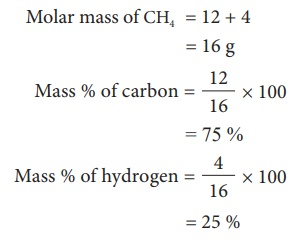Home | | Science 10th Std | Percent Composition

# Percent Composition

The percentage composition of a Â­compound represents the mass of each element present in 100 g of the compound.

PERCENT COMPOSITION

So for, we were dealing with the number of entities present in a given substance. But many times, the information regarding the percentage of a particular element present in a compound is required.

The percentage composition of a Â­compound represents the mass of each element present in 100 g of the compound.

Let us understand the percentage Â­composition of oxygen and hydrogen by taking the example of H2O. It can be calculated using the formulaThis percentage composition is useful to determine the empirical formula and molecular formula.

Example 1: Find the mass percentage Â­composition of methane (CH4).Tags : Atoms and Molecules , 10th Science : Chapter 7 : Atoms and Molecules
Study Material, Lecturing Notes, Assignment, Reference, Wiki description explanation, brief detail
10th Science : Chapter 7 : Atoms and Molecules : Percent Composition | Atoms and Molecules